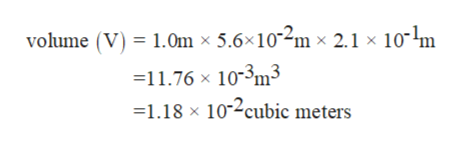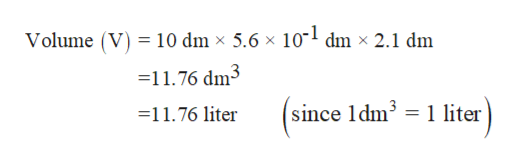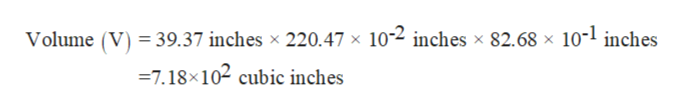# A rectangular solid measures 1.0 m by 5.6 cm by 2.1 dm. Express its volume in cubic meters, liters, cubic inches, and cubic feet

Question
135 views

A rectangular solid measures 1.0 m by 5.6 cm by 2.1 dm. Express its volume in cubic meters, liters, cubic inches, and cubic feet

check_circle

Step 1

The volume of the rectangle can be calculated by the formula,

Volume (V) = length (l) × width (b) × height (h)  ………(1)

Given that,

Length of the rectangular solid is 1.0 m

Width of the rectangular solid is 5.6 cm

Height of the rectangular solid is 2.1 dm

First, convert all the sides in the unit of meter by using the following conversion data as follows:

1 cm = 10-2 m

Therefore, 5.6 cm is equal to 5.6×10-2 m

1 dm = 10-1 m

Therefore, 2.1 dm is equal to 2.1×10-1 m

Now calculate the volume of the rectangular solid by using the equation (1) as follows:

Thus the volume of the rectangular solid is 1.18 ×10-2 cubic meters.help_outlineImage Transcriptionclosevolume (V) 1.0m x 5.6x102m x 2.1 x 101m 11.76 x 10-3m3 1.18 x 102cubic meters fullscreen
Step 2

Now the volume is to be calculated in liters.

As it is known as,

1 liter is equal to 1 dm3

Convert all the units in dm3 as follows:

Since 1 m is equal to 10 dm

1 cm is equal to 10-1 dm

Therefore, 5.6 cm is equal to 5.6×10-1 dm

Now calculate the volume of the rectangular solid by substituting these values in equation (1) as follows:

Therefore, the volume of the rectangular solid in liter is 11.76 literhelp_outlineImage TranscriptioncloseVolume (V 10 dm x 5.6 x 10" dm x 2.1 dm =11.76 dm3 since 1dm3 1 liter =11.76 liter fullscreen
Step 3

Now volume is to be calculated in cubic inches.

Convert all the given lengths in inches.

1 m is equal to 39.37 inches

Since 5.6 cm is equal to 5.6 × 10-2 m

Therefore 5.6 cm in inches is,

(5.6 × 10-2 × 39.37) inches

=220.47 × 10-2 inches

Now convert 2.1 dm in inches as follows:

2.1 dm is equal to 2.1 × 10-1 m.

Or 2.1 dm is ...help_outlineImage TranscriptioncloseVolume V39.37 inches x 220.47 x 102 inches x 82.68 x 10-1 inches =7.18x102 cubic inches fullscreen

### Want to see the full answer?

See Solution

#### Want to see this answer and more?

Solutions are written by subject experts who are available 24/7. Questions are typically answered within 1 hour.*

See Solution
*Response times may vary by subject and question.
Tagged in

### Chemistry